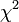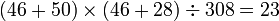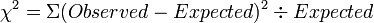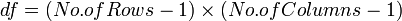# Hypothesis Testing

## Overview

In Comparative Studies, often the best way to analyse and report the results is to use confidence intervals. However, statistical hypothesis tests are still widely used in scientific work and thus, we need to consider how these tests work. Most statistical analyses involve comparisons (usually between treatments and procedures or between groups of subjects).

## Types of Hypotheses

The medical hypothesis is the basis of the statistical hypotheses (i.e. null hypothesis H0 and alternative hypothesis H1).

### Medical Hypothesis

The medical hypothesis is the starting point. It is statement that presents an idea of association between variables. For example, progressive polyarthritis (PAP) is associated with the HLA-DR4 antigen.

### Statistical Hypotheses

#### Null Hypothesis H0

A null hypothesis states that there is no association between the variables of interest. For example, there is no association of PAP with HLA-DR4.

#### Alternative Hypothesis H1

An alternative hypothesis states that there is a real association between the variables of interest. For example, PAP is associated with HLA- DR4.

## Fourfold & Contingency Tables

Fourfold and Contingency Tables are used to calculate observed frequencies, expected frequencies, chi-squared statistic$\chi^2$ and degree of freedom (df).

A chi-squared test is used to determine whether there is an association between two variables, which may be:

• qualitative
• discrete quantitative
• continuous quantitative (where values have been grouped)

Data from two such variables may be arranged in a contingency table. The categories for one variable define the rows, and the categories for the other variable define the columns. Individuals are assigned to the appropriate cell. When the table only has two rows or two columns, the table is called a four-fold table.

#### Observed and Expected Frequencies

Observed frequencies are simply the observed number of subjects that apply to each cell. The expected frequencies for each cell are the numbers that we would expect to find if the null hypothesis was true (i.e. no association). To calculate he expected frequency for each cell, we use the observed frequencies. For a given cell, the sum of the cell's row multiplied by the sum of the cell's column, divided by the total number of subjects n. See the example below:

Observed frequencies in the sample of 308 patients divided according to presence of PAP and HLA-DR4

PAP HLA-DR4 Total
Present Absent
Present 46 28 74
Absent 50 184 234
Total 96 212 308

Expected frequencies in the sample of 308 patients divided according to presence of PAP and HLA-DR4

PAP HLA-DR4 Total
Present Absent
Present 23 51 74
Absent 73 161 234
Total 96 212 308

You can see that for the first cell, the expected value was calculated as follows:$(46+50)\times(46+28)\div308=23$

#### Chi-Squared Statistic$\chi^2$

For the alternative hypothesis to be true (i.e. presence of association), the observed and expected frequencies, should be close together, any discrepancy being due to random variation. The best way to this is a chi-squared test, where the observed and expected values are compared by this formula:$\chi^2 = \Sigma (Observed-Expected)^2 \div Expected$

For the example above, we would like to perform the statistical test with 5% level of significance. The test result is$\chi^2$=43.61. In order to interpret this chi-squared statistic, the number of degrees of freedom (df) is needed. For a contingency table, df is calculated by the formula:$df = ( No. of Rows -1 )\times ( No. of Columns-1 )$

For the example table, df=1. Using a reference table which shows the percentage points of the$\chi^2$ distribution, we can see that 43.62 is greater than 3.84 (the critical value for 5% level of significance). Please see this chi-squared distribution reference table:

Thus the probability is less than 5% that such a large observed difference could have risen by chance. Thus we can reject the null hypothesis. The use of chi-squared test is not confined to nominal and ordinal data but also can be used for continuous variables hat have been categorised.